# Number of Protons Atomic Number Number of Protons

• Slides: 48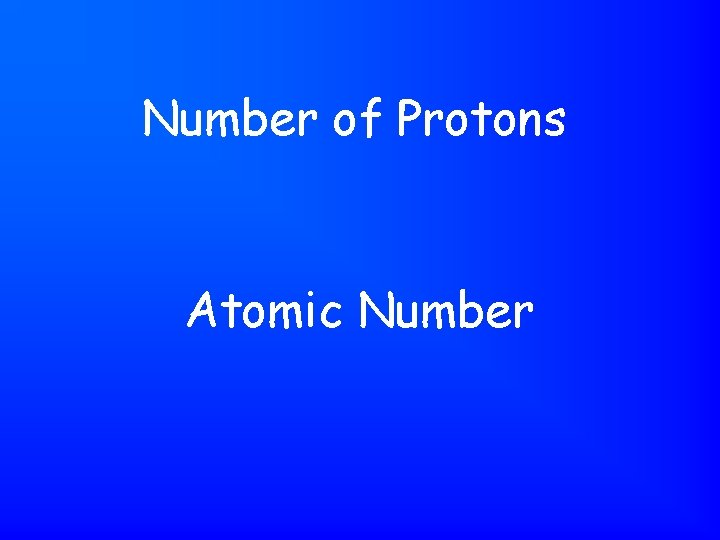Number of Protons Atomic Number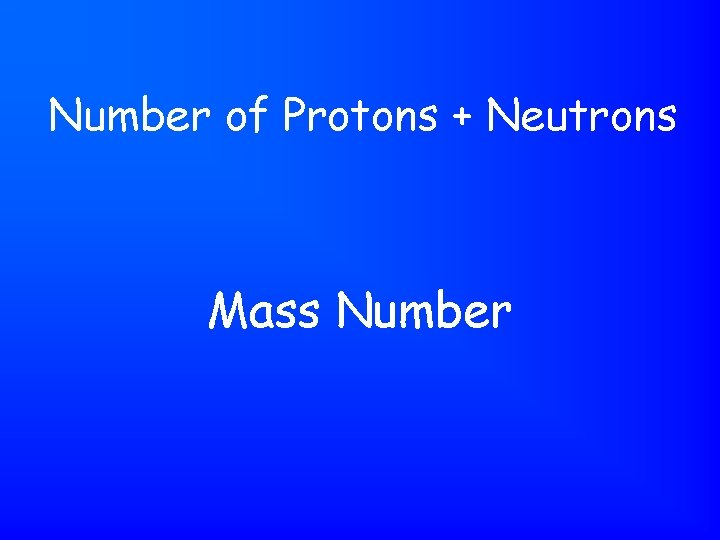Number of Protons + Neutrons Mass Number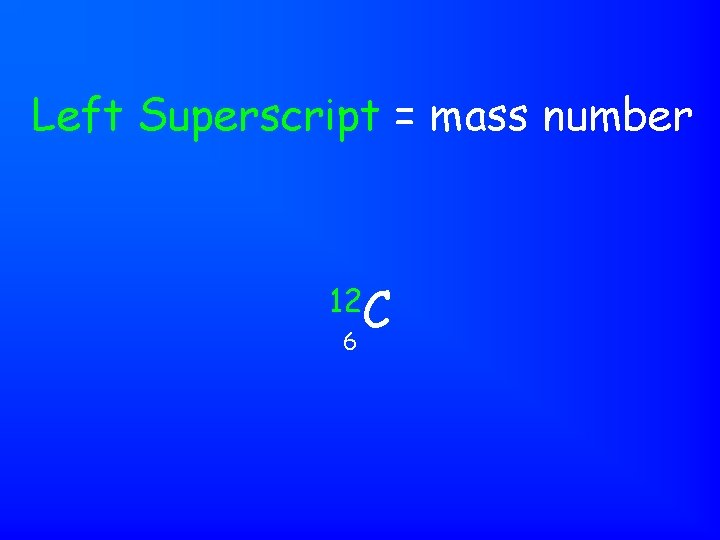Left Superscript = mass number 12 C 6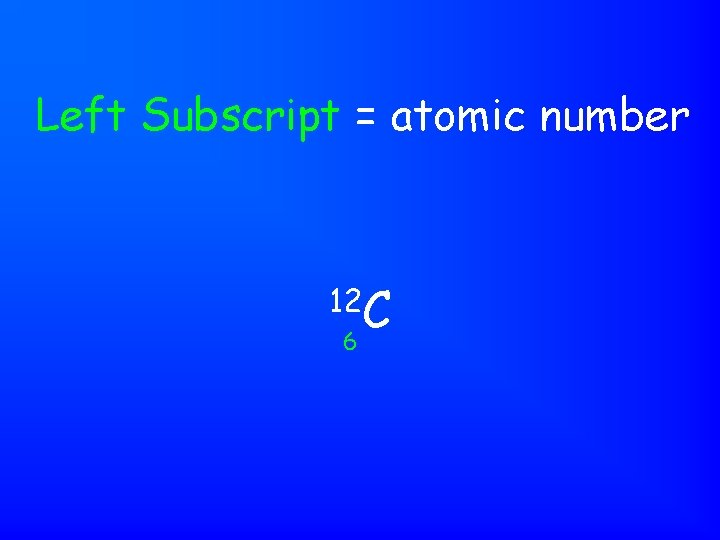Left Subscript = atomic number 12 C 6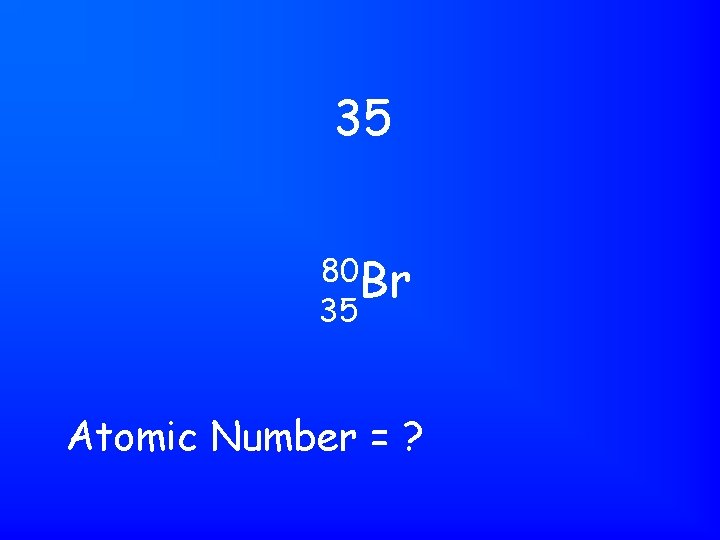35 80 Br 35 Atomic Number = ?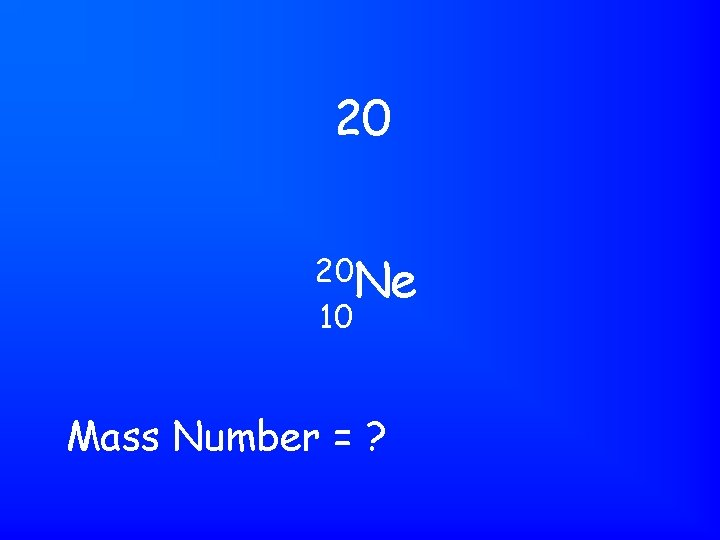20 20 Ne 10 Mass Number = ?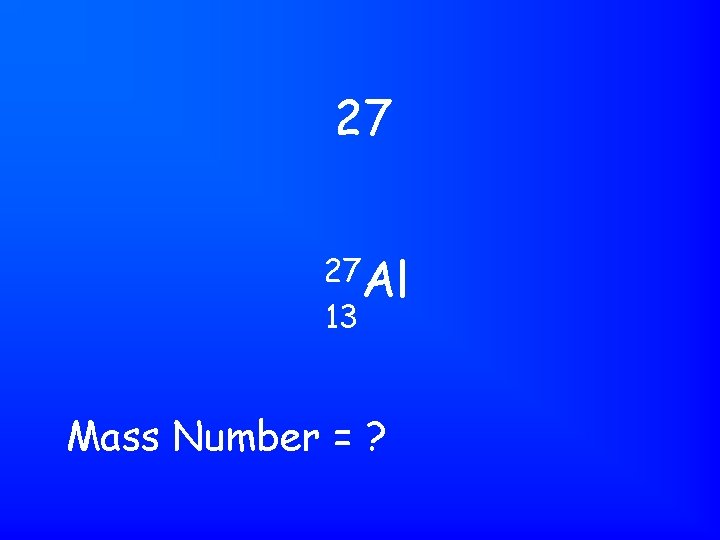27 27 Al 13 Mass Number = ?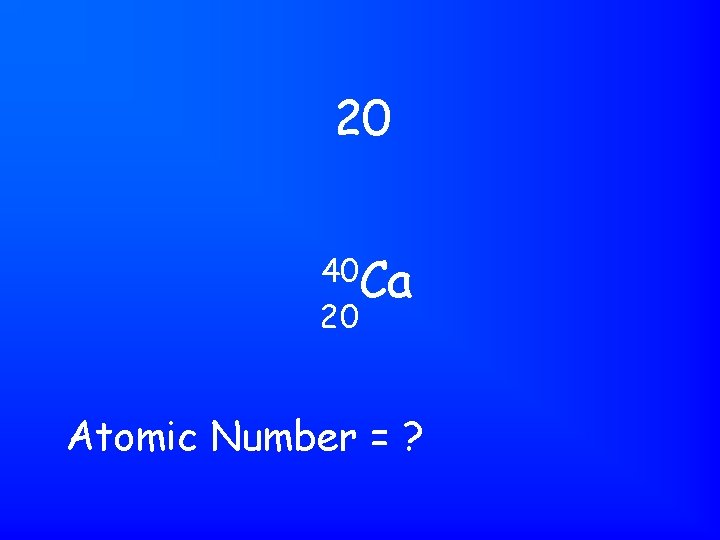20 40 Ca 20 Atomic Number = ?Neutral atoms have the same number of electrons and protons. # of electrons in a neutral atom?Atoms of the same element with a different # of neutrons IsotopeCharge = +1, mass = 1 amu, location = inside nucleus Characteristics of ProtonCharge = 0, mass = 1 amu, location = inside nucleus Characteristics of NeutronCharge = -1, mass = 1/1836 amu or 0. 0005 amu, location = outside nucleus Characteristics of Electron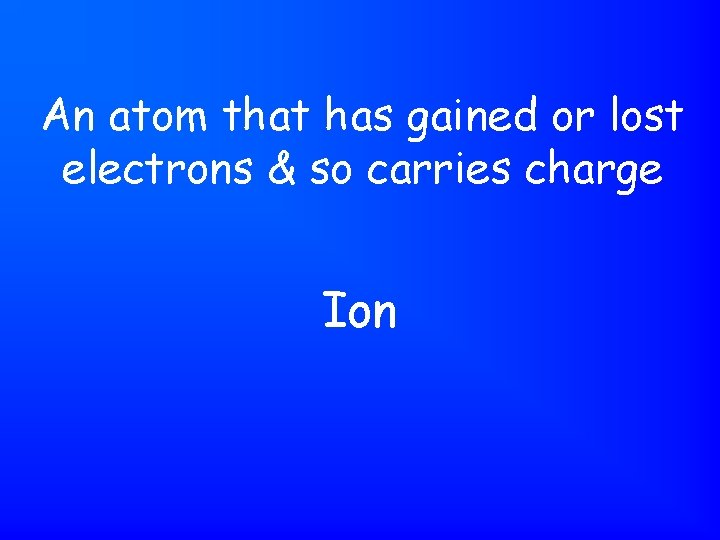An atom that has gained or lost electrons & so carries charge Ion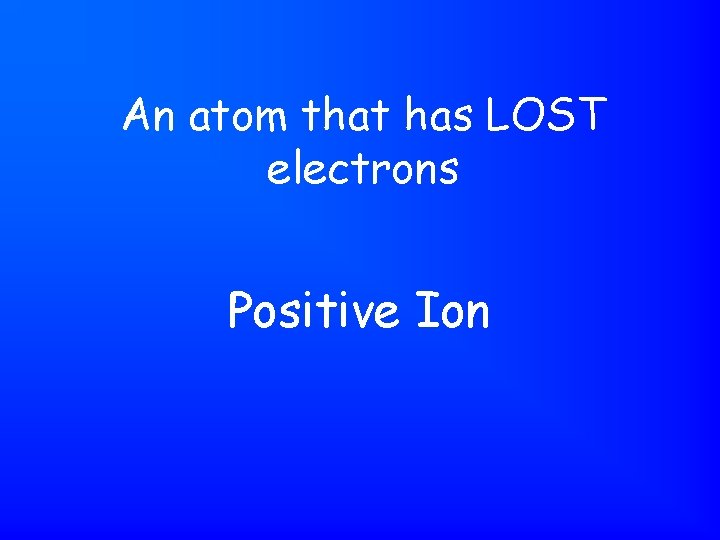An atom that has LOST electrons Positive Ion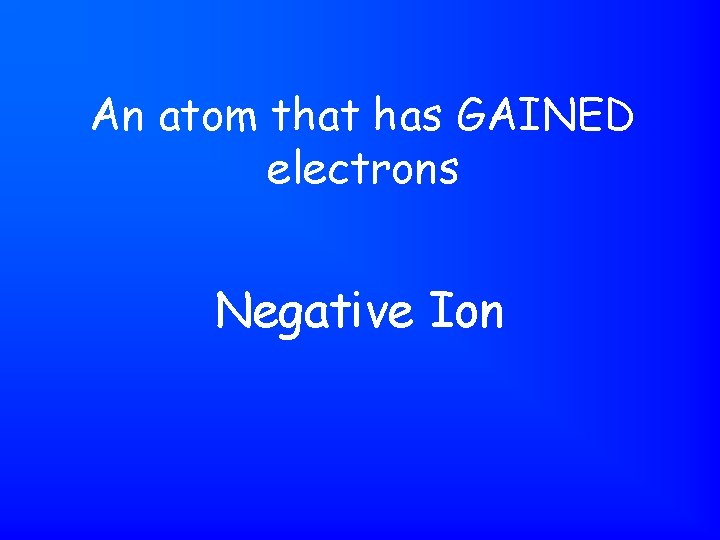An atom that has GAINED electrons Negative Ion# protons - # electrons Charge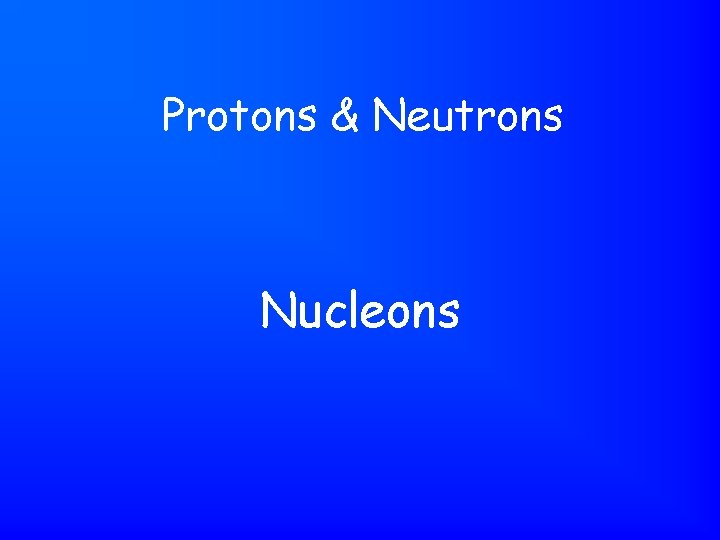Protons & Neutrons Nucleons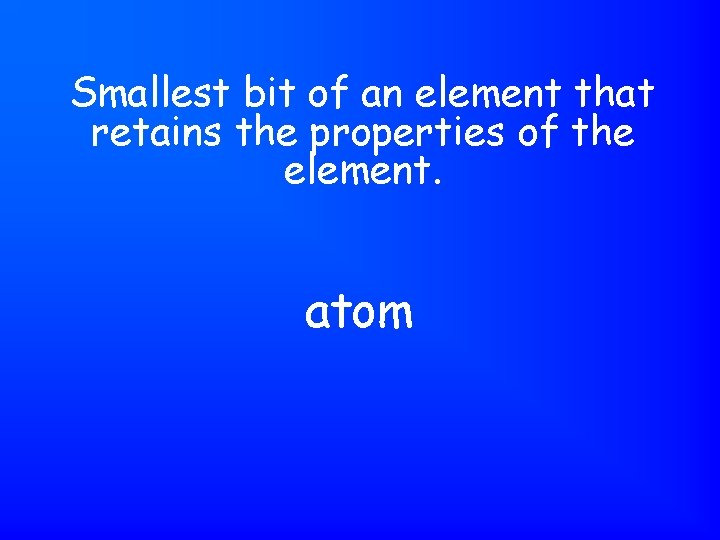Smallest bit of an element that retains the properties of the element. atom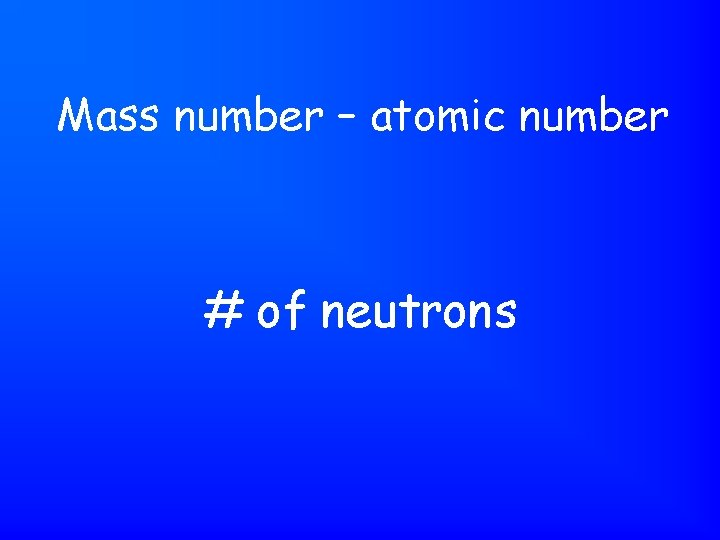Mass number – atomic number # of neutrons8 neutrons 6 protons 6 electrons 14 C 6 # of neutrons = ? # of protons = ? # of electrons = ?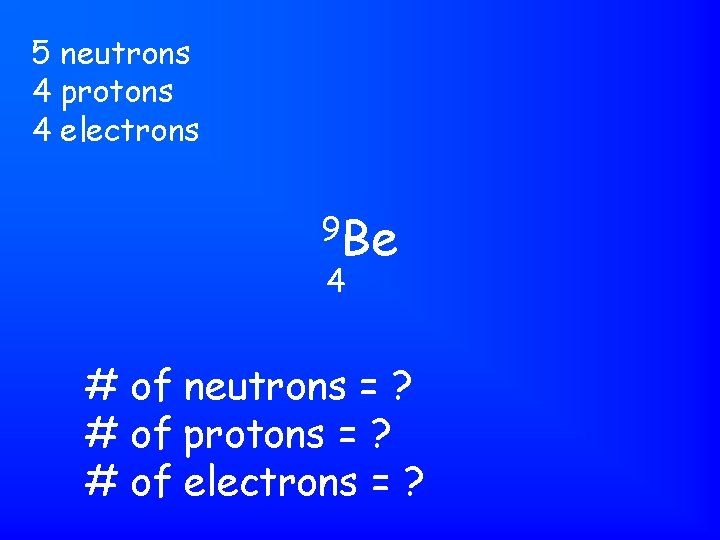5 neutrons 4 protons 4 electrons 9 Be 4 # of neutrons = ? # of protons = ? # of electrons = ?22 neutrons 18 protons 18 electrons 40 Ar 18 # of neutrons = ? # of protons = ? # of electrons = ?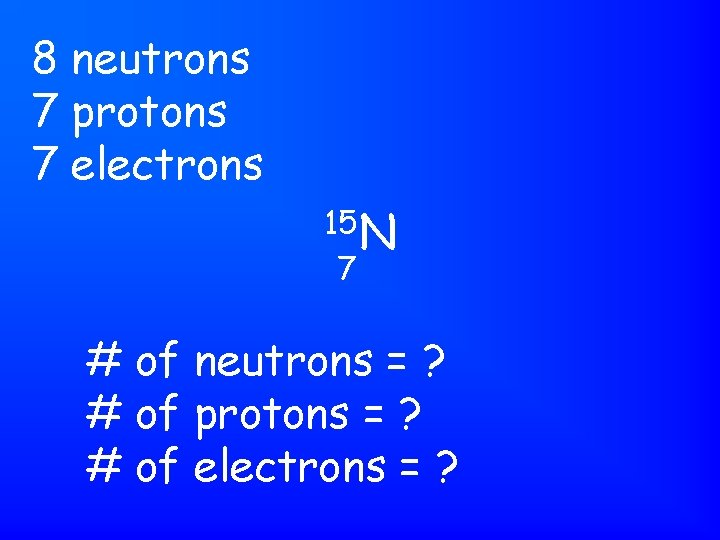8 neutrons 7 protons 7 electrons 15 N 7 # of neutrons = ? # of protons = ? # of electrons = ?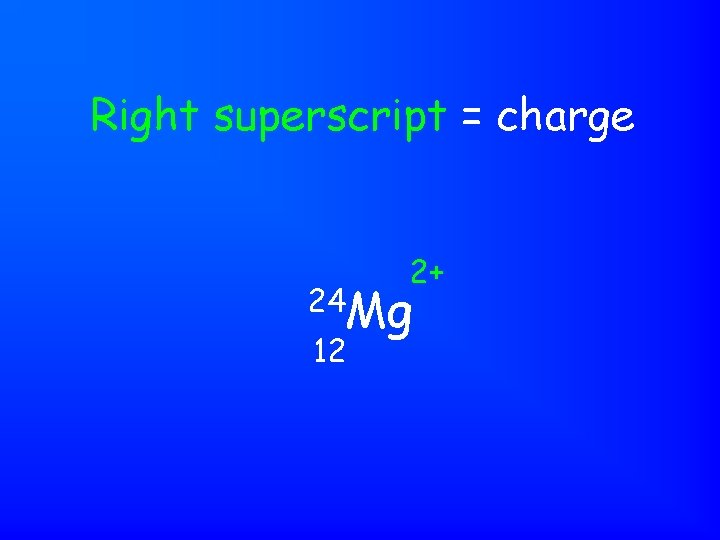Right superscript = charge 2+ 24 Mg 12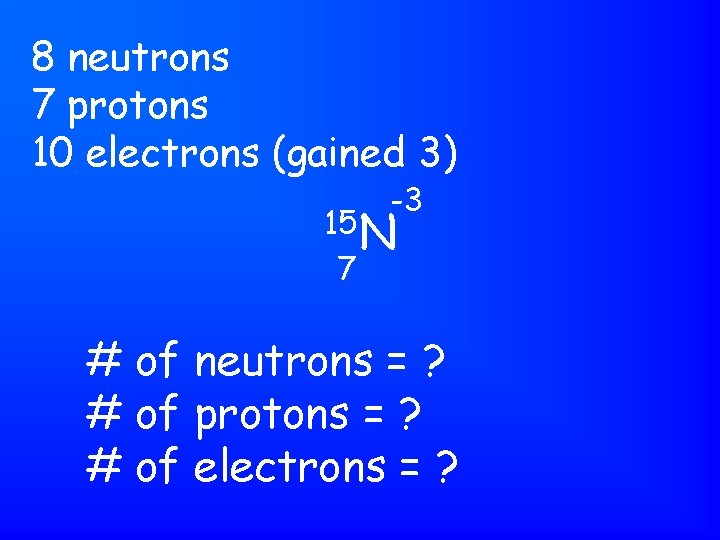8 neutrons 7 protons 10 electrons (gained 3) -3 15 N 7 # of neutrons = ? # of protons = ? # of electrons = ?10 neutrons 9 protons 10 electrons (gained 1) -1 19 F 9 # of neutrons = ? # of protons = ? # of electrons = ?8 neutrons 8 protons 10 electrons (gained 2) -2 16 O 8 # of neutrons = ? # of protons = ? # of electrons = ?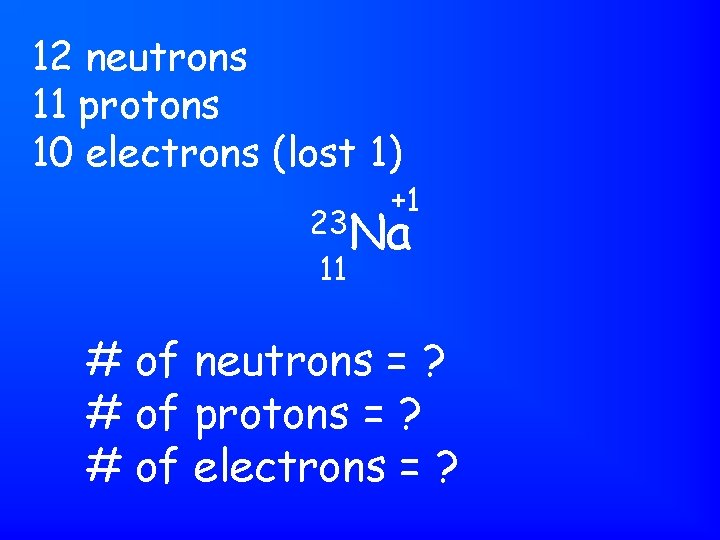12 neutrons 11 protons 10 electrons (lost 1) +1 23 Na 11 # of neutrons = ? # of protons = ? # of electrons = ?12 neutrons 12 protons 10 electrons (lost 2) +2 24 Mg 12 # of neutrons = ? # of protons = ? # of electrons = ?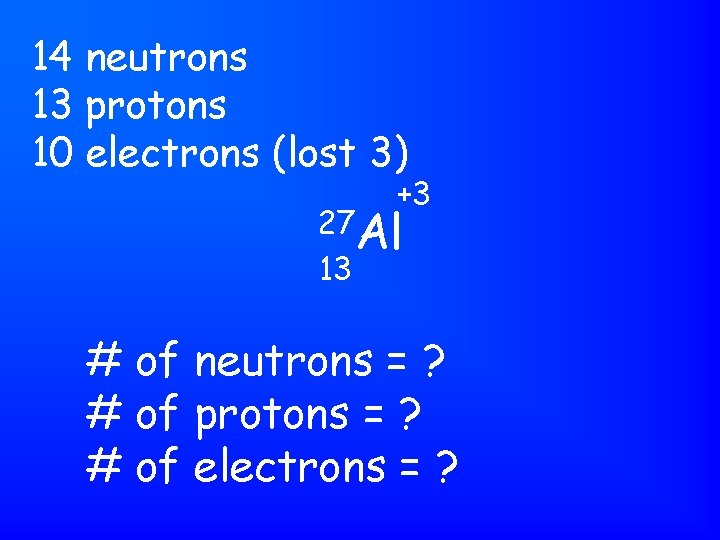14 neutrons 13 protons 10 electrons (lost 3) +3 27 Al 13 # of neutrons = ? # of protons = ? # of electrons = ?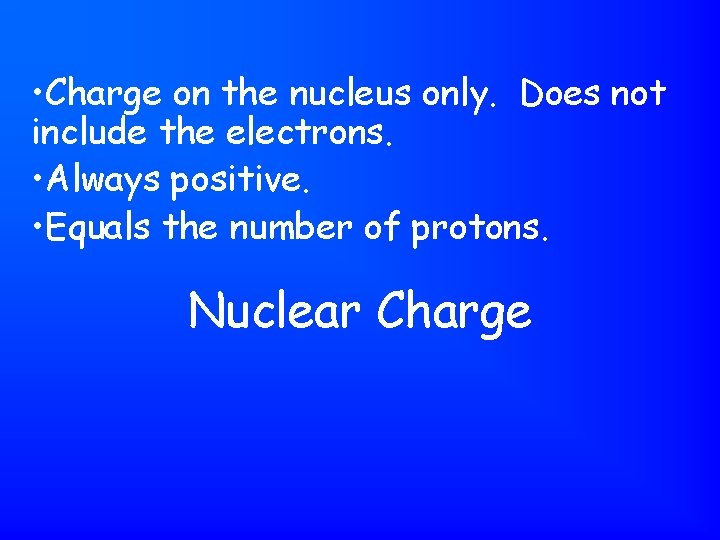• Charge on the nucleus only. Does not include the electrons. • Always positive. • Equals the number of protons. Nuclear ChargePositive ion CationNegative ion AnionBilliard Ball Model Dalton’s ModelAtoms are hard, round, indivisible. They are solid & uniform throughout. Dalton’s modelPlum Pudding Model Thomson’s Model - + + -+ -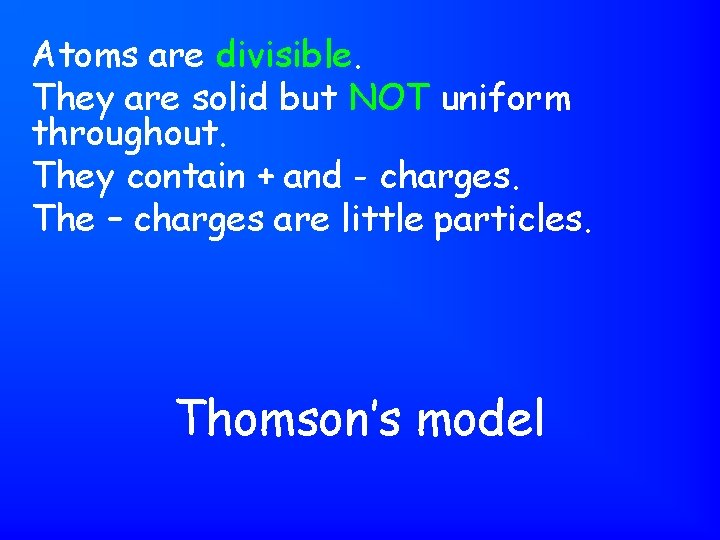Atoms are divisible. They are solid but NOT uniform throughout. They contain + and - charges. The – charges are little particles. Thomson’s model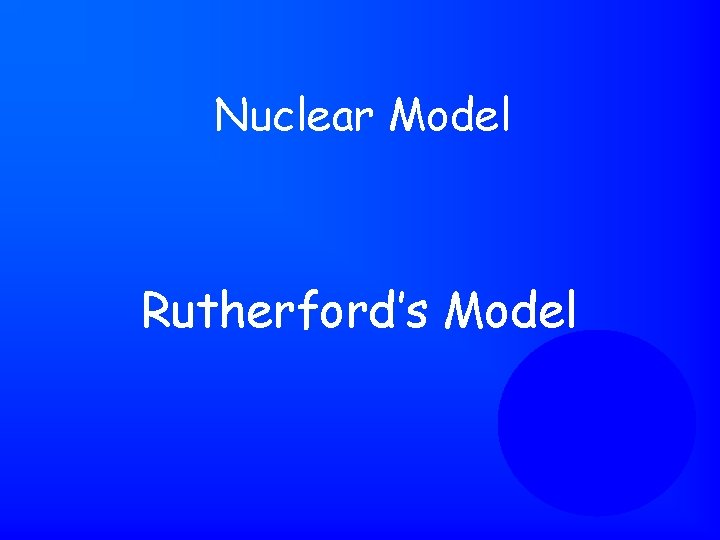Nuclear Model Rutherford’s Model - + -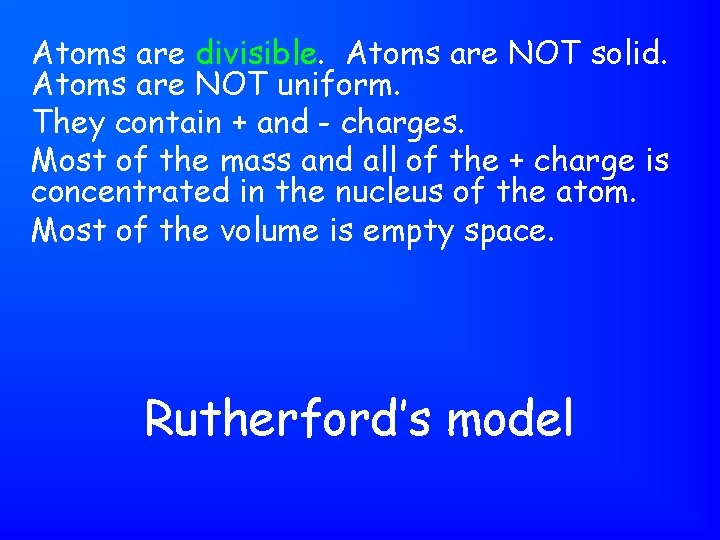Atoms are divisible. Atoms are NOT solid. Atoms are NOT uniform. They contain + and - charges. Most of the mass and all of the + charge is concentrated in the nucleus of the atom. Most of the volume is empty space. Rutherford’s model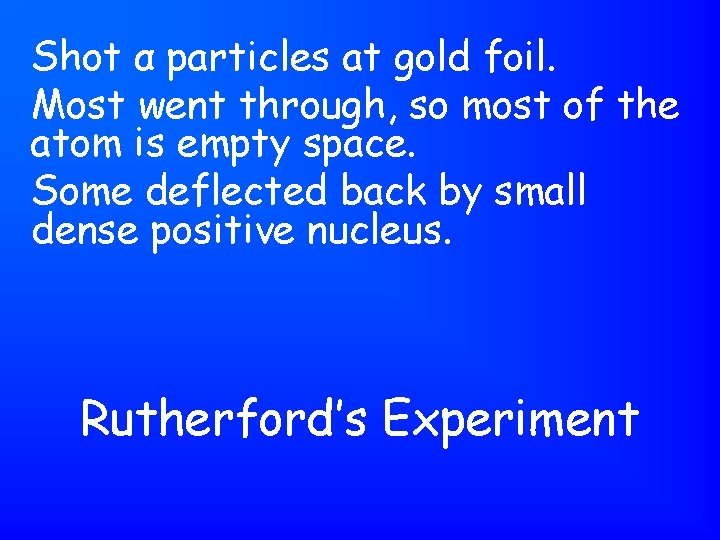Shot α particles at gold foil. Most went through, so most of the atom is empty space. Some deflected back by small dense positive nucleus. Rutherford’s ExperimentPlanetary Model Bohr’s ModelThe mass of the entire atom: includes protons, neutrons, electrons. Expressed relative to the mass of a C-12 atomic mass1 atomic mass unit 1/12 the mass of a C-12 atom. or The C-12 atom has a mass of 12. 000. . . atomic mass units. atomic mass unit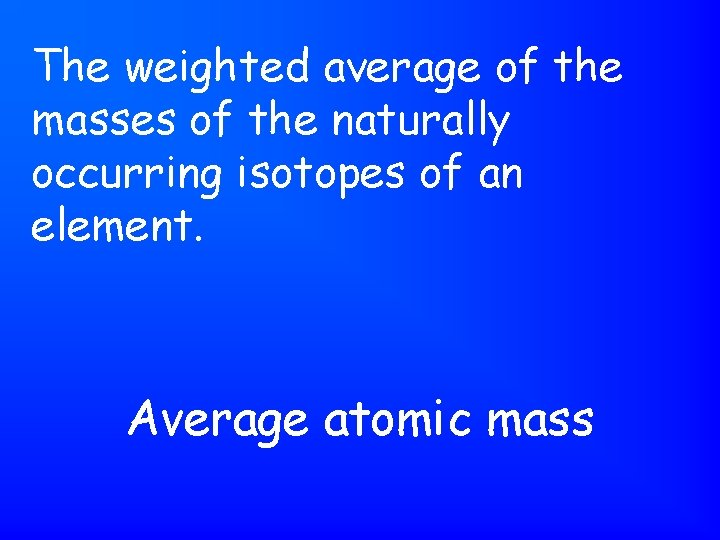The weighted average of the masses of the naturally occurring isotopes of an element. Average atomic mass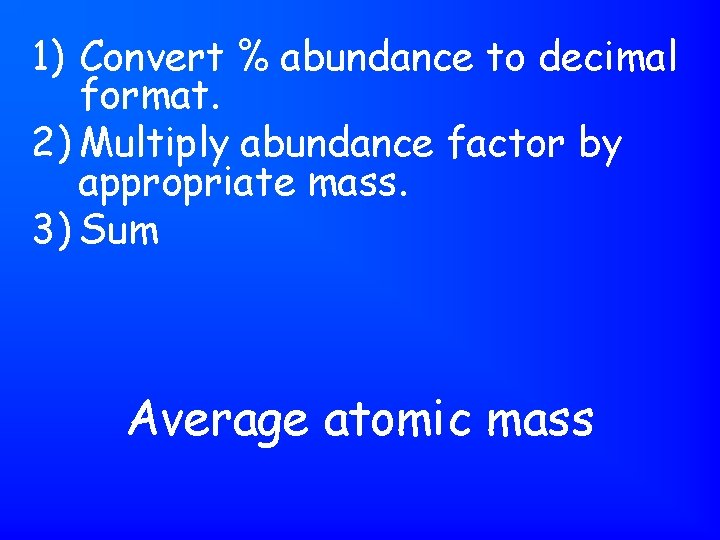1) Convert % abundance to decimal format. 2) Multiply abundance factor by appropriate mass. 3) Sum Average atomic mass1) Final answer must be between the highest & lowest masses. 2) Final answer will be closest to mass of most abundant isotope. Quick check on average atomic mass calculation.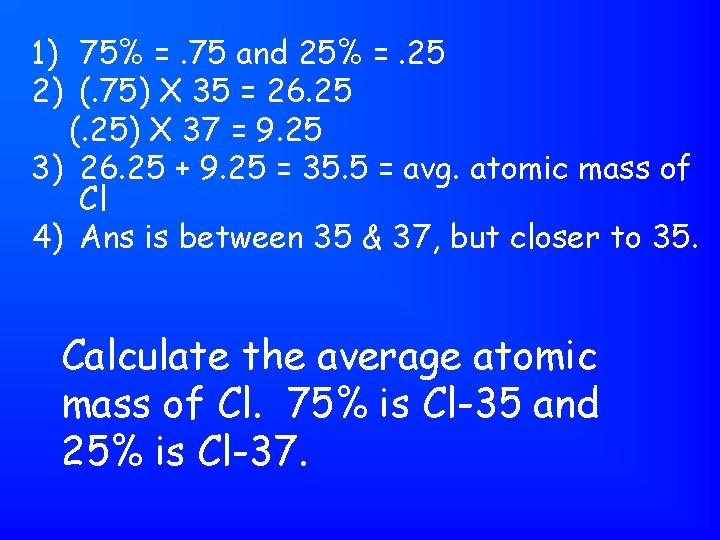1) 75% =. 75 and 25% =. 25 2) (. 75) X 35 = 26. 25 (. 25) X 37 = 9. 25 3) 26. 25 + 9. 25 = 35. 5 = avg. atomic mass of Cl 4) Ans is between 35 & 37, but closer to 35. Calculate the average atomic mass of Cl. 75% is Cl-35 and 25% is Cl-37.